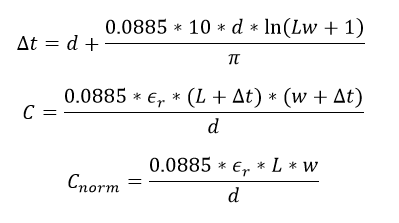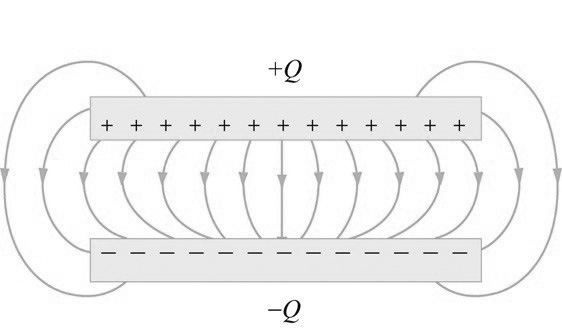# Edge Effect of Rectangular Parallel Plate Capacitor Calculator

This CalcTown calculator calculates the edge effect of a rectangular parallel plate capacitor.

The goal of this problem is to explore, in a crude sense, the correction to the capacitance C of a parallel plate capacitor due to edge effects.

#### Result

pF
pFWhere,

L = length of the rectangular parallel plates

w = width of the rectangular parallel plates

d = dielectric thickness

Δt = increase in effective length and width due to edge effect

εr = relative permittivity

Edge Effect in a Capacitor

If d << √A, then on the length scale of d, near the edge of the capacitor, the capacitor looks like parallel semi-infinite plates separated by a distance d. Let us suppose that the upper plate is held at potential ϕ0/2, and is located at a distance y = d/2 above the x-axis; the lower plate is held at potential −ϕ0/2 and is located at y = −d/2. Suppose that δ0 is the change in capacitance per unit length associated with such a scenario.

We can approximate

C/ε = A/d + Lδ

where, L is the perimeter of the capacitor plate.

To compute the edge effects, we use conformal mapping. Consider the transformation,

z=dw/ϕ0 + de2Πw/ϕ0/2Π

Here z represents the spatial coordinate, and w the complex electric potential.

Thus we can write

δ = 1/2Π * log(√A/d) + δ0

where δ0 is an unknown constant (independent of d or A).